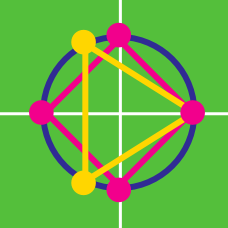Algebra

# Roots of Unity: Level 3 Challenges

If $w \not= 1$ is an $n$-th root of unity, then find the value of

$1+w+w^2+w^3+\cdots +w^{n-1}$

Evaluate

$\cos \frac{ \pi}{7} - \cos \frac{ 2\pi }{7} + \cos \frac{ 3 \pi}{7} .$

$\huge x^{1729}+x^{-1729}$

Find the value of the above expression if $x + \frac 1 x = 1$

If for $z \in \mathbb{C} \space ,$ $z+\dfrac{1}{z}=2 \cos 6°.$ Then find the value of $\left( z^{1000}+\frac{1}{z^{1000}} \right).$

Given $z^2+z+1=0,$ find the value of

$\left(z+\dfrac{1}{z} \right)^2+\left(z^2+\dfrac{1}{z^2}\right)^2+\left(z^3+\dfrac{1}{z^3} \right)^2+\cdots+\left(z^{21}+\dfrac{1}{z^{21}}\right)^2.$

×